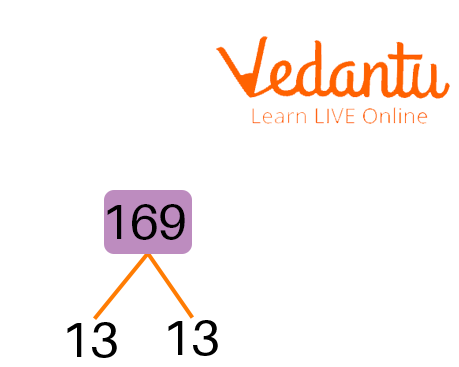Courses
Courses for Kids
Free study material
Offline Centres
More

# Factors of 169Last updated date: 25th Nov 2023
Total views: 118.5k
Views today: 4.18k## What is the Factor of 169?

Natural numbers that can divide the number 169 into equal parts are known as its factors. The product of two natural numbers is 169, then the 2 numbers are the required factors. 169 is the perfect square of 13, since, $13\times 13=169$ and 13 is an odd prime number. Also, 169 is a composite number. The pair factors of the number 169 can be expressed in positive form or negative form. For example, the pair factors of the number 169 are represented as $\left ( 1,169 \right )$ or $\left ( -1,-169 \right )$. We multiply the pair of negative numbers, such as multiplying $-1$ and $-169$, and we will get the original number.

## Finding the Factors of 169

A factor of 169 is another integer that can divide the integer without leaving any remainder i.e. zero remainder. Therefore, the way you find and list all of the factors of 169 is to go through every number up to and including 169 and check which numbers result in an even quotient (that means no decimal place).

Below are all of the factors of 169:

$169 \div 1 = 169$

$169 \div 13 = 13$

$169 \div 169 = 1$

All of these factors can be used to divide 169 by and get a whole number. The full list of positive factors for 169 are $1, 13$, and $169.$

## Prime Factors of 169 : Prime Factorisation

Prime factorisation or prime factor decomposition is the process of finding which prime numbers can be multiplied together to get the original number.Factor Tree of 169

The prime factors are: $13 \times 13$ or also written as $\left \{ 13,13 \right \}$

Written in exponential form: $13^{2}$

## Pair Factors of 169

Two numbers, when multiplied together, equal 169 make for the pair factors of the number. The list of all the 169 factor pairs is provided below. We can see that when we multiply all the factor pairs together, we get 169.

$1 \times 169 = 169$

$13 \times 13 = 169$

$169 \times 1 = 169$

## All Factors of 169

The factors of 169 are numbers that when divided by 169, leave zero as remainders. Those that can divide 169 exactly i.e. factors are $1, 13, 169$.

 Factors Pair Factors Prime Factorisation 1, 13, 169 (1,169), (13,13) $169=13 \times 13=13^2$

## Solved Examples

Example 1: There are 169 fruits to be divided among 13 children. Using the factors of 169, calculate the number of fruits each child gets.

Solution: From the factors of the number 169, we know that $169 = 1 \times 13 \times 13$

$\therefore 169 = 13 \times 13$

Therefore, when there are $13$ children, each child will get $13$ fruits.

Example 2: Find the highest common factors of 13 and 169.

Solution: The prime factorisation of $13$ and 169 is given by

$13 = 1 \times 13$

$169 = 1 \times 13 \times 13$

To find the HCF, we multiply the factors which are common to both $13$ and 169.

$HCF\left ( 13,169 \right )=1 \times 13 = 13$

## Practise Questions

1. What is the LCM of 169 and $196$?

1. 33153

2. 33124

3. 22341

4. 32431

Ans: B

2. What is the square root of 169?

1. 13

2. 14

3. 12

4. 16

Ans: A

## Conclusion

In this article, we learnt that the factors of 169 are numbers that, when divided by 169, leave zero as remainders. The number 169 has a total of 3 factors: $1, 13, 169$. Its prime factors are $13 \times 13$. The factors in pair for 169 are $\left ( 1,169 \right )$, $\left ( 13,13 \right )$ and $\left ( 169,1 \right )$. You will now be able to factor 169, we hope this article was helpful.

## FAQs on Factors of 169

1. Is 169 a prime number?

No, 169 is not a prime number. Depending on the number of factors, it contains—it may have only two factors or more—a number can be categorised as prime or composite. Exactly two factors are required for a number to be categorised as a prime number. 169 is not a prime number because it has more than two factors, including $1$, $13$, and 169. In other words, the fact that 169 has more than two factors makes it a composite number.

2. Is 169 a perfect square?

Any number that can be expressed as the product of an integer by itself or as the second exponent of an integer is referred to as a perfect square. Just like we factorise a regular number, we can factorise a perfect square number. It can be expressed as the sum of a single number. The result of the prime factorisation of 169 is $13 \times 13$. $13$ is the square root of 169. Therefore, 169 is a perfect square.

3. How do you find the prime factors of any number?

he prime factors are the prime numbers that divide the given number. Any number can be written as a product of prime numbers. The prime number is divisible only by $1$ and the number itself. If $‘a’$ and $‘b’$ are the only prime factors of a number $‘x’$, then $‘x’$  can be written in the form: $a^{n} \times b^{m}$ where n  and m are natural numbers. The prime factors of a number are calculated by dividing the given number with prime numbers starting from $2,3…$ and continuing up to half of the number. The prime numbers which completely divide the number are the prime factors of the number.